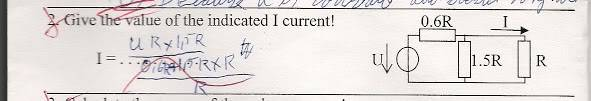# Easy question: find the current

## Homework Statement

Can somebody please explain to me what i did wrong here? Got exam tomorrow and it would probably be usefull to know.. Thxaxb = a*b/(a+b)

## The Attempt at a Solution

First i tried finding the voltage over 1.5R x R.. Then divide that voltage over R to find the current over R... For some strange reason this is wrong. Plz someone help me to understand why..

## Answers and Replies

treat the 1.5R and R as parallel. then in series with the 0.6R to get the overall resistance. current going through 0.6R = voltage/overall R. Then the same current split into the 1.5R and R, calculate.

nice, thx a lot. Now i got further :)

However if u would be able to point out what i assumed wrong in my first attempt itd be apreciated as well, so i dont happen to do a similar mistake again

hmm... its like really basic. just get familar with kirchoff's v and i law. u'll be fine